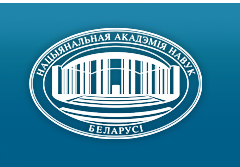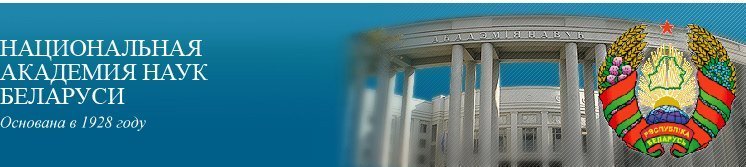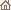Главная Новости Об академии Деятельность академии Члены академии Организации Издания академии Мероприятия Контакты Электронные обращения Справочная информация Карта сайта

Известия Академии наук Беларуси. Сер. физ.-матем. наук, 1997, No.2/ Издания академии / Научные журналы

Известия Академии наук Беларуси. Сер. физ.-матем. наук, 1997, No.2Известия Академии наук Беларуси СЕРИЯ ФИЗИКО-МАТЕМАТИЧЕСКИХ НАУКИздатель: Беларуская навука, Минск, Республика Беларусь

Номер 2, 1997СОДЕРЖАНИЕ

МАТЕМАТИКА

Ignatenko M.V., Yanovich L.A.
Using Hankel's transformation for construction of operator interpolation formulas. pp. 5--10

Summary: The Newton interpolation formulas for differentiable and nondifferentiable operators defined on the set of functions for which the Hankel transformation and its inverse exist are constructed.

Rovba Ye.A.
Interpolation of piecewise analytical functions by rational ones. pp. 11--15

Summary: Interpolation of piecewise analytical functions by rational ones are studied.

Levina T.I., Matsevich D.A., Sinkevich D.V.
On the first moment of an estimate of the Markovian chain parameters, based on aggregated data. pp. 16--21

Summary: An asymptotic formula for calculation of the expectation of transitional probabilities of a homogeneous Markovian chain for the polynomial scheme is obtained.

Morozova I.M.
Approximations of zero by the scalar product of integer-valued vectors and an analytical vector-function. pp. 22--25

Summary: New estimates for the order of approximation of zero by values of analytical functions of a special form is obtained.

Zhuk Ye.Ye.
Asymptotic optimality of the generalized likelihood function based decision rules of cluster analysis. pp. 26--31

Summary: Generalized likelihood function based decision rules of cluster analysis are considered. Conditions of their optimality are found by means of the risk asymptotic expansion method.

Zuev N.M.
The convergence rate for the distribution of sum maximum of sequence of random variables with uniformly strong intermixing. pp. 32--35

Summary: Let {Xn} be a strictly stationary symmetrical and j be mixing sequence of random variables, E X1 = 0, Sn = ånj=1Xj, s2n = D Sn, limn®µsn = µ, Sn = max1£ k £ n Sk, x+ = max(0, x). Theorem. Suppose that there exist constants R > 0, b > 0, 0 < s £ 1, C0 > 0 such that j(t) £ R exp{-bt}, E |X1|2+s £ C0. Then for any e > 0 there exists C = C(R, b, e, s, C0) < µ such that supx | P(Sn/sn £ x) - Ö (2/p) ò0x+ exp{-y2/2} dy | £ Cn-g+e, where g = min (1/15, s/(4s+2)).

Vasiliev A.F., Vasilieva T.I.
On the three times factorable finite solvable groups). pp. 36--39

Summary: Schunck classes X and local formations Á of finite solvable groups with the condition that X contains each group G = AB = BC = CA, where AÎXÇÁ, BÎXÇÁ and CÎX, is investigated.

Medvedev G.A., Serdiukov E.V.
Properties of maximum likelihood estimates for software reliability models. pp. 40--45

Summary: Nine software reliability models are investigated to find out properties of solutions of the maximum likelihood equations for estimates of unknown parameters. Numerical results are also given.

Muha V.S.
Differentiating functions of symmetric matrices. pp. 46--53

Summary: Techniques for matrix differentiation in the case where the variable is symmetric matrix of order 2 is developed. The main differentiation rules are given. Derivatives of the moat widespread functions of the matrix variable are obtained.

Savchuk V.P., Savenkov V.A., Vyarvilskaya O.N.
Vibration of a transport line during the motion of a load stream. pp. 54--57

Summary: Stationary vibrations of a transport line with infinite number of spans are investigated. The supporting ability of the line, along with a rigid frame, is provided by strongly strained flexible elements. The dynamic deflection of span is found, and the maximum value of its stationary components is determined.

Krushevski A.E., Boiko N.Ya.
Compressing an elastic free parallelepiped under the end-walls action of normal movings of the wedge-shaped diagram. pp. 58--60

Summary: Problem about equilibrium of an elastic parallelepiped under the action of vertical wedge-shaped movings on the facet z = ± h/2, with the other facets free of load, is solved on the basis of a modification of the Kantorovich -- Vlasov variational method.

ФИЗИКА

Komarov L.I., David O.I. Obeahon
A linear model for the polaron in the strong coupling region. pp. 61--65

Summary: The operator transformation technique is developed to obtain the energy levels and effective mass of polarons in the strong coupling approximation. The model consists in the linearity of equations of motion for quantum operators and boils down to the fact that the approximating hamiltonian is self-conforming in respect to its parameters and it has a quadratic form which can be fully diagonalized. In our approximation the average amplitude of oscillations of electron in the ground and first excited states is considered to be small and all the terms in approximating hamiltonian containing the product of more than two quantum operators are neglected.

Grinchik N.N., Muchinski A.N., Tsurko V.A.
Simulation of electrical phenomena in the distributed systems. pp. 66--70

Summary: The electromagnetic field equations, reduced to the telegraph equation, and the corresponding diffusion equation are considered to describe diffusion-electric phenomena. Simulating the charge of homogeneous medium is carried out.

Korshunov F.P., Lastovski S.B.
Influence of annealing radiation defects on the breakdown voltage of silicon p-n-junctions. pp. 71--74

Summary: An increase of the breakdown voltage (measured at 300 K) after isochronal annealing (353--673 K) of silicon p--n-junctions irradiated with 4 MeV electrons was observed within temperature ranges 357--473 and 533--593 K. Changes of the breakdown voltage at 393--473 K depend on electron fluency. This phenomenon is related to peculiarities of annealing E-centers.

Gusakov V.Ye.
Quantum chemical simulation of the local structure and electronic spectrum of small clusters of boron nitride and silicon. pp. 75--78

Summary: Quantum chemical simulation of small clusters of NB and Si have been performed. The defect structure of hydrogen on the bond B -- N is determined. Photo fragmentation section of small Si clusters as a function of the size is calculated and compared with experimental data.

Brinkevich D.I., Vabishchevich S.A., Petrov V.V.
Thermal donors in silicon grown in nitrogen environment. pp. 79--82

Summary: Processes of thermal donor generation in silicon grown by the Czochralski method in the nitrogen atmosphere (N -- Si) are studied. It is shown that acceleration of thermal donor generation takes place in N -- Si.

Gea I.A., Kuzmich G.V., Romanov I.M.
Study of properties of the electrochromic thin films of sodium-tungsten bronzes. pp. 83--87

Summary: We investigate properties of electrochromic thin films of sodium-tungsten bronzes with composition NaxWO3, which were produced with the help of electron beam evaporation. The element surface composition analysis of thin films of sodium tungsten bronzes has been carried out. Changes in their electron structure depending on parameters of the production process have been studied.

Novysh B.V., Dorozhkin N.N., Gololobov Ye.M., Anishchik V.M.
On calculating the anharmonic components of the potential of electron-ion interaction. pp. 88--91

Summary: A simple model is developed to calculate the non-linear in atomic displacement components of electron-lattice interaction. These contributions give rise to violation of electron-ion scattering selection rules valid in the rigid Muffin-tin approximation.

Akimov A.I., Akimova T.M., Blizniuk L.A., Savchuk G.K.
Investigation of influence of the compaction on the density of PZT-42-1 under high pressures. pp. 92--95

Summary: Influence of high external pressure on the density of PZT-42-1 is investigated.

Gololobov Ye.M., Chaplanov A.M., Mazurenok O.G.
Formation of the HTSC Y-Ba-Cu-O(F)-system films prepared by the method of electron beam layer-after-layer deposition. pp. 96--100

Summary: Phase and structure transformations as well as electrochemical properties of Y-Ba-Cu-O(F)-system films, prepared by the electron beam layer-after-layer deposition method is investigated.

Zaporozhets E.V., Kasparov K.N., Malyshev S.A., Tyavlovskaya E.A.
Ohmic contacts with gallium arsenide of p-type. pp. 101--103

Summary: Experimental results for specific resistance of ohmic contacts depending on their formation conditions are presented. Metal-semiconductor interaction during the fusing-in process is investigated with the use of the XPS method.

Gololobov E.M., Prytkova N.A., Tomilo Zh.M., Turtsevich D.M., Ladutko N.F.
High-temperature superconducting coatings of substrates based on Ni-Cu alloys. pp. 104--106

Summary: Preparing and properties of the YBCO and BSCCO coatings of substrates based on Ni-Cu alloy are reported. The properties of the obtained coatings are compared with those of the coatings on nickel substrates.

Shiripov V.Ya., Fedosenko G.N., Yemelianov V.A., Fedosenko N.N., Chizhik S.A.
Study of the structure of diamond-like carbon films. pp. 107--108

Summary: Study of the diamond-like films by means of the atomic-force microscopy and the Raman spectroscopy is presented.

ИНФОРМАТИКА

Tuzikov A.V., Margolin G.L.
Symmetry measures of convex sets. pp. 109--114

Summary: Measures of rotation and reflection symmetries for compact convex sets are introduced and investigated. Appropriate symmetrization transformations are used to transform the original sets to symmetric ones. The symmetry measures are defined to be the ratio of volumes (the Lebesgue measure) of the original set and the corresponding symmetric set. For the case of rotation symmetry, a generalization of the Minkowski symmetric set for a cyclic group of rotations is used as a symmetrization transformation. For the case of reflection symmetry, Blaschke's symmetrization is investigated. The problem of fast computation of symmetrization transformation is discussed. Since the Minkowski addition of two sets is reduced to the convolution of their characteristic functions in case of the discrete plane, the Fast Fourier Transformation can be applied to the fast computation of symmetrization transformation.

Katkov V.L., Kolesnik A.M., Kuznetaov G.P., Mikutski V.S., Oleinik L.F.
On one approach to simulation of the spatial structure of ecology systems. pp. 115--119

Summary: Interactive system BELFOR/BELFOR-S, which is intended for simulation of the forest stand structure and its dynamics are presented. The techniques of point processes is used to investigate and simulate the stand spatial structure. Some approach is exploited that enables one to link the statistical parameters of the stand spatial structure with a dynamical model of forest. The system simulates emergence, growth and death of trees on a site with an arbitrary specified type of an initial spatial pattern.

Rimski G.V.
Root-locus method for robust optimization of performance. pp. 120--123

Summary: Methods of general root locus theory are used for solving the problem of robust optimization of performance. Equations of functional root loci are derived. Theorems of robust stability of control systems with uncertain plants are proved.

Tihonenko O.M., Zirko O.F.
Multi-line queuing systems with random length demands, restricted memory and random time restrictions. pp. 124--127

Summary: We consider the queueing systems M|M|n|m in which each demand is characterized by a random length, the service time does not depend on the length, the sum of demand lengths presented in a system is bounded with a constant and the queueing time or the waiting time is restricted with an exponential random variable. For such systems, distribution of the amount of demands in the stationary state is determined.

АННОТАЦИИ ДЕПОНИРОВАННЫХ СТАТЕЙ

Dolgih M.N., Krot A.M., Romanovskaya N.A.
Data compression for digital images, based on discrete orthogonal transforms in the basis of finite group characters. pp. 128--128

Monastyrny P.I., Shanko D.V.
On convergence and optimization of the method of invariant boundary conditions embedding for nonlinear difference equations. pp. 128--129

Metelski N.N., Tarasevich A.Ye.
Effective enumeration of maximal pointed convex cones. pp. 129--129

Savenko B.C., Ostrikov O.M.
Strength fields near the infinite dislocation ladder. pp. 129--129

Milman V.A.
Extensions of real-valued functions under restriction on the variation rate. pp. 130--130

Trush N.N., Mirskaya E.I.
A modified periodogram on each segment of an observation partition. pp. 130--130

Mirskaya E.I.
A modification of the finite Fourier transform on each segment of an observation partition. pp. 130--130

In Belarusian Mathematical Society. pp. 131--135

ХРОНИКА

Rakovich A.G., Savik N.P.
A new achievement in design and production automation. pp. 136--138/ Издания академии / Научные журналы / К началу страницы

Разработана и поддерживается Николаем Н. Костюковичем. Последнее обновление: 7 ноября 2006 г.
Создана при участии Игнатия И. Корсака
Копирайт © 1998-2006 Национальная академии наук Беларуси
Копирайт © 1997 Издательство "Беларуская навука"